# Use the given graph off over the interval (0, 6) to find the followingelchatosarapage 2021-11-20 Answered
Use the given graph off over the interval (0, 6) to find the following.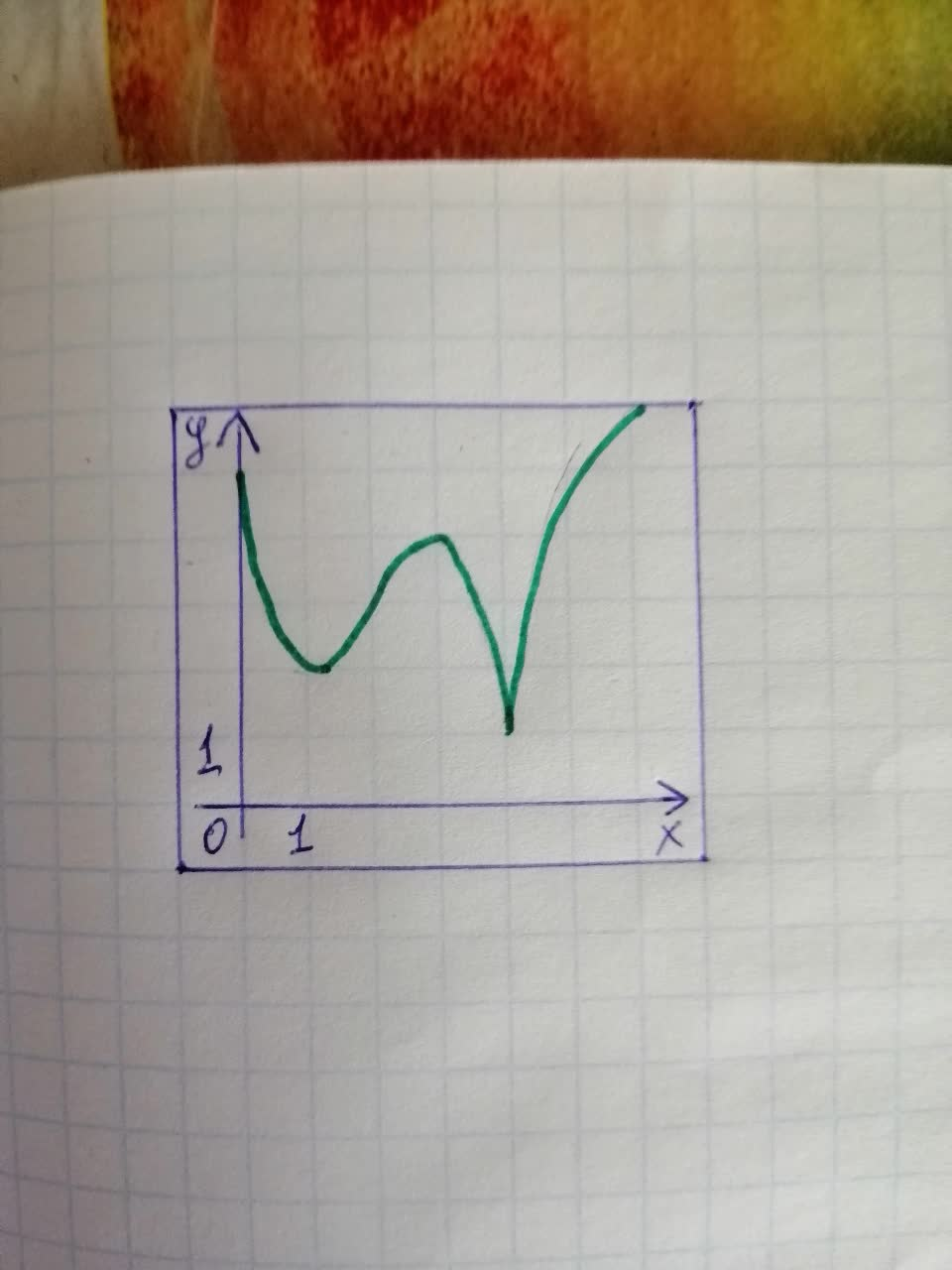a) The open intervals on whichfis increasing. (Enter your answer using interval notation.)
b) The open intervals on whichfis decreasing. (Enter your answer using interval notation.)
c) The open intervals on whichfis concave upward. (Enter your answer using interval notation.)
d) The open intervals on whichfis concave downward. (Enter your answer using interval notation.)
e) The coordinates of the point of inflection. $$\displaystyle{\left({x},\ {y}\right)}=$$

• Questions are typically answered in as fast as 30 minutes

### Plainmath recommends

• Get a detailed answer even on the hardest topics.
• Ask an expert for a step-by-step guidance to learn to do it yourself.Clara Clark
Step 1
a) $$\displaystyle{\left({1},\ {3}\right)},\ {\left({4},\ {6}\right)}$$
b) $$\displaystyle{\left({0},\ {1}\right)},\ {\left({3},\ {4}\right)}$$
c) $$\displaystyle{\left({0},\ {2}\right)}$$
d) $$\displaystyle{\left({2},\ {4}\right)},\ {\left({4},\ {6}\right)}$$
e) $$\displaystyle{\left({2},\ {3}\right)}$$
###### Have a similar question?Froldigh
Step 1
a) The open intervals on which $$\displaystyle{f}$$ is increasing.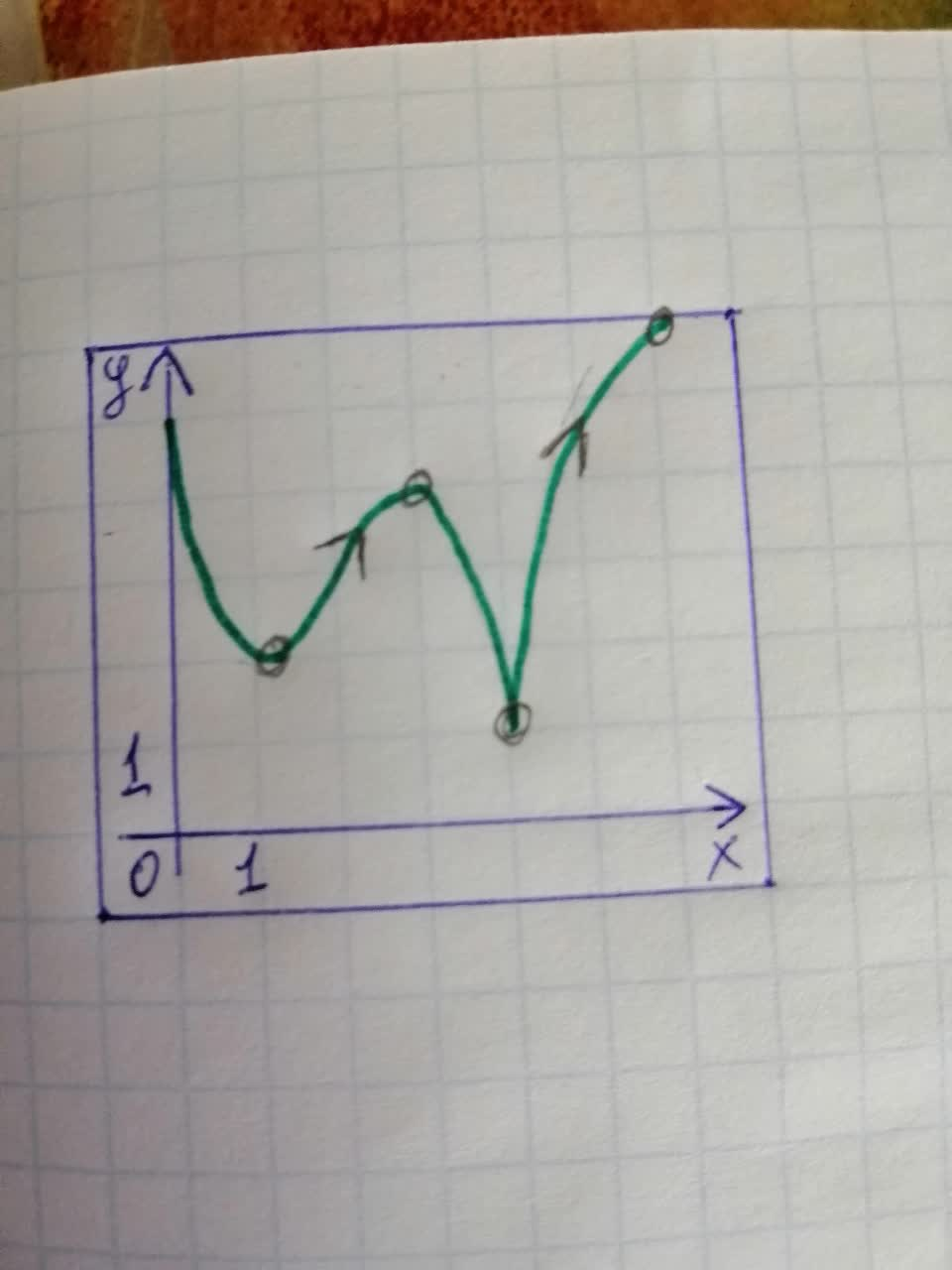From the above graph it is observed that (observed the arrows and the black dots), the function increasing in the interval $$\displaystyle{\left({1},\ {3}\right)}$$ and $$\displaystyle{\left({4},\ {6}\right)}$$ as they have the tangents with positive slope (positive slope means the tangents slightly bends towards right).
b) The open intervals on which $$\displaystyle{f}$$ is decreasing.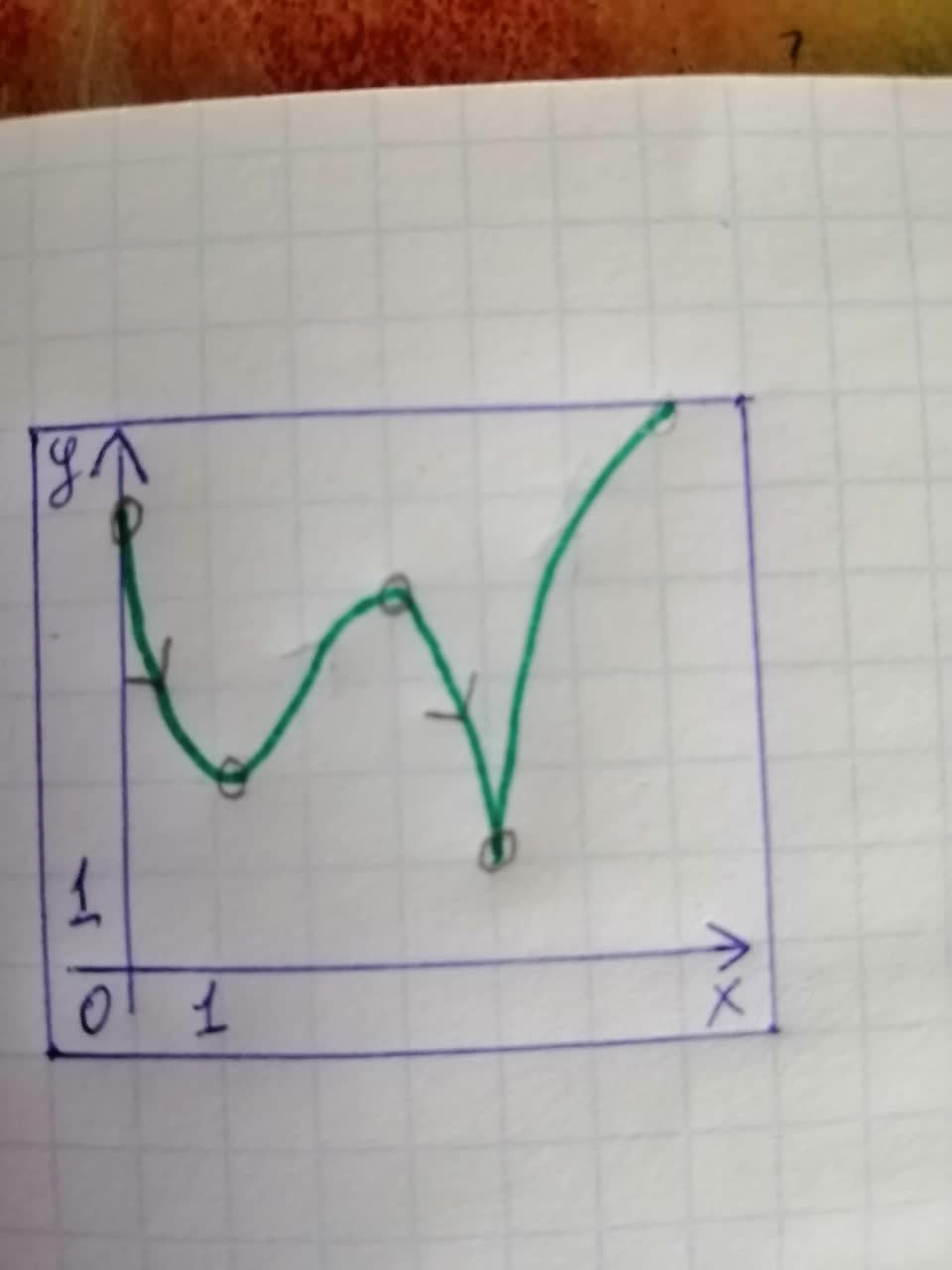From the above graph it is observed that (observe the arrows and the black dots), the function increasing in the interval $$\displaystyle{\left({0},\ {1}\right)}$$ and $$\displaystyle{\left({3},\ {4}\right)}$$ as they have the tangents with negative slope (negative slope means the tangents slightly bends towards left).
c) The open intervals on which $$\displaystyle{f}$$ is concave upward.
The function $$\displaystyle{f{{\left({x}\right)}}}$$ is concave up for the interval in which $$\displaystyle{f}{''}{\left({x}\right)}{>}{0}$$
If the curve of the function $$\displaystyle{f{{\left({x}\right)}}}$$ always remains above the tangent lines for every point in the interval $$\displaystyle{I}$$, we say that the curve is concave upward on that interval.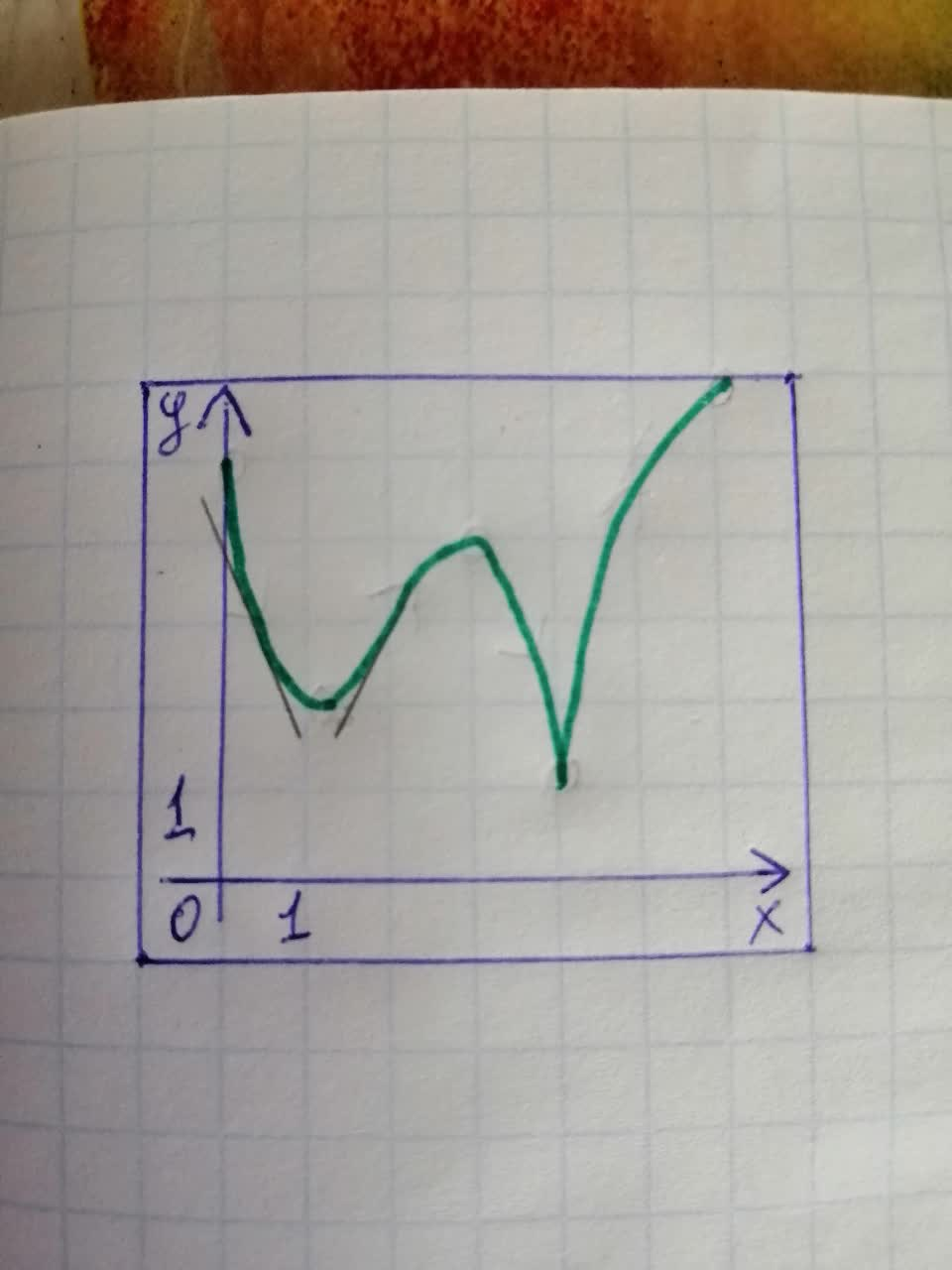From the graph it is observed that the curve remain above the tangent lines in the interval $$\displaystyle{\left({0},\ {2}\right)}$$ hence it is concave up in the interval $$\displaystyle{\left({0},\ {2}\right)}$$
d) The function $$\displaystyle{f{{\left({x}\right)}}}$$ is concave down for the interval in which $$\displaystyle{f}{''}{\left({x}\right)}{<}{0}$$</span>
If th curve of the function $$\displaystyle{f{{\left({x}\right)}}}$$ always remains below the tangent lines for every point in the interval $$\displaystyle{I}$$, we say that the curve is concave down on that interval.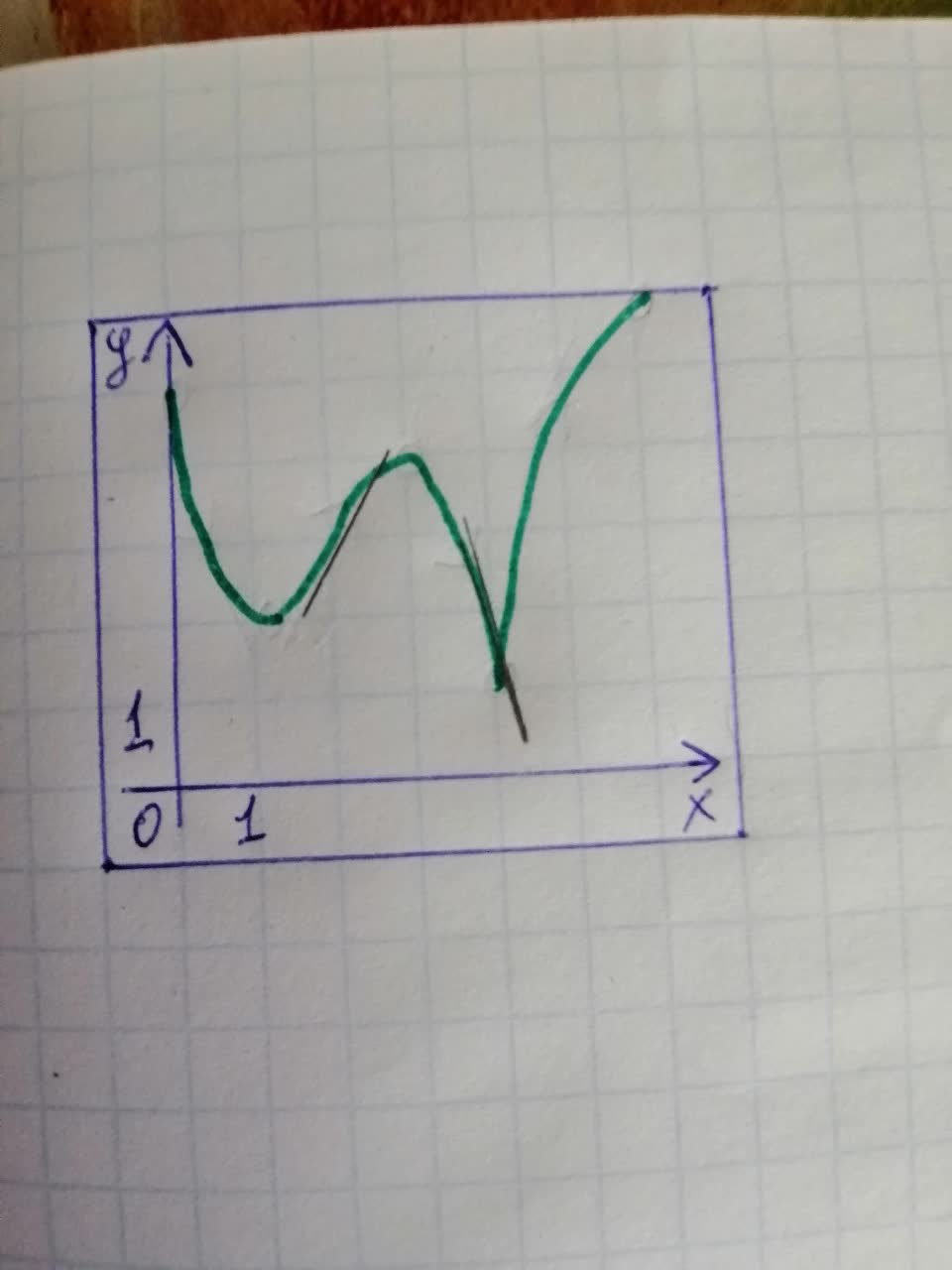From the graph it is observed that the curve remain below the tangent lines in the interval $$\displaystyle{\left({2},\ {4}\right)}$$ hence it is concave down in the interval $$\displaystyle{\left({2},\ {4}\right)}$$
e) From the above parts we have that the function is concave up in the interval $$\displaystyle{\left({0},\ {2}\right)}$$ and concave down in the interval $$\displaystyle{\left({2},\ {4}\right)}$$ which means the concavity of the function is changing at the point 2.
This follows that the infection point for the given function is at $$\displaystyle{x}={2}$$